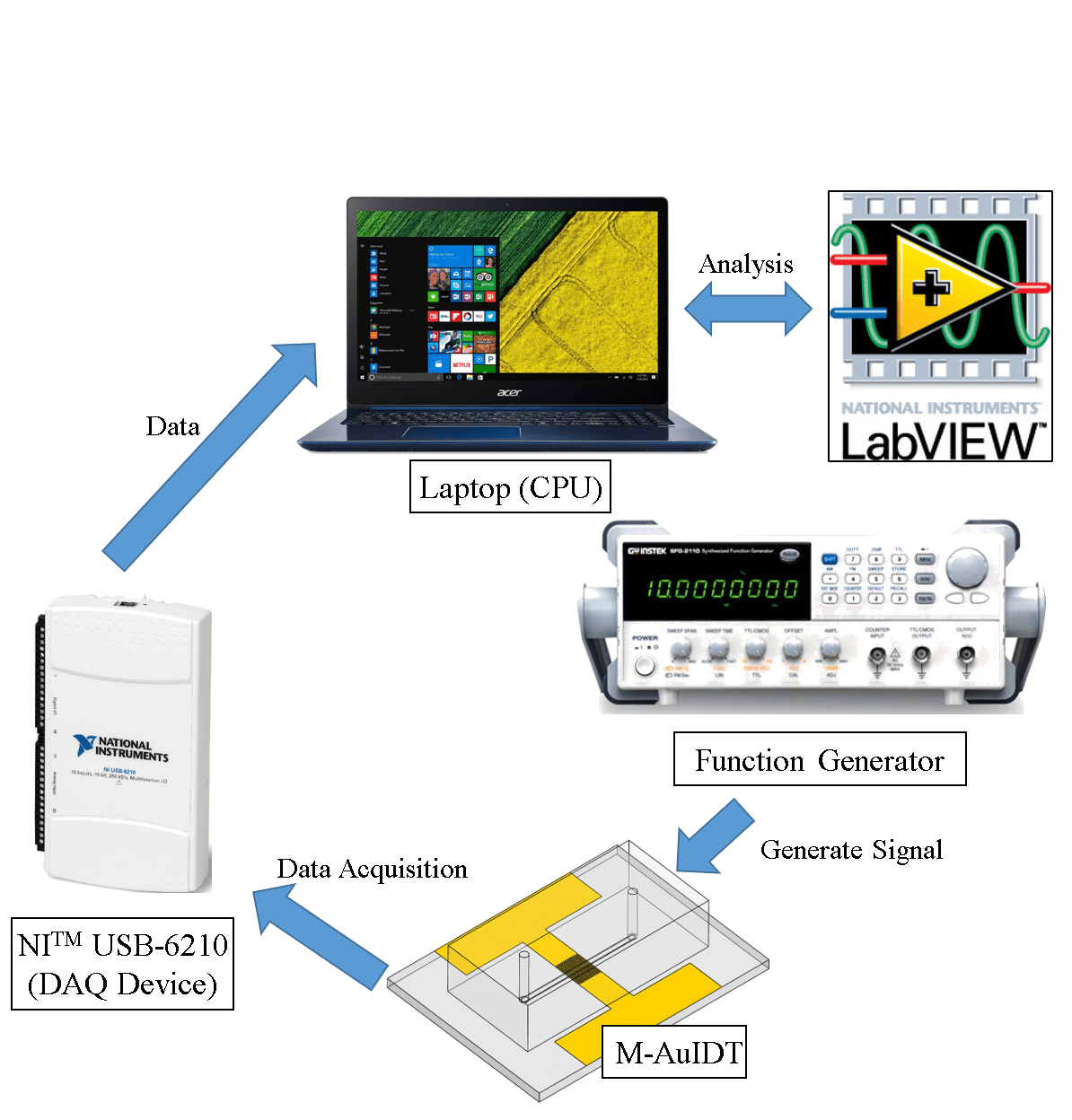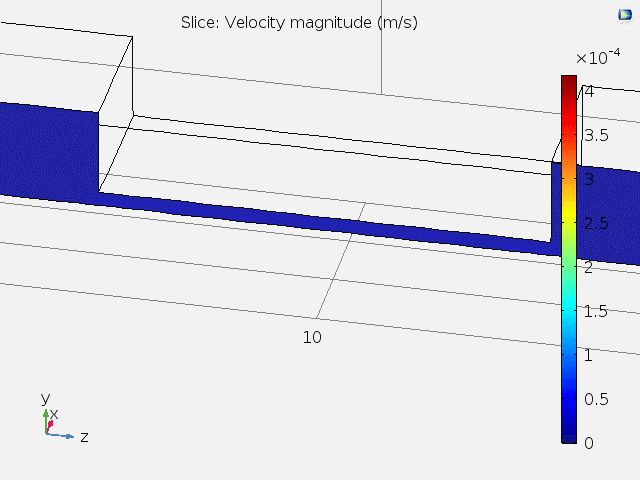## Electrochemical and Biological Simulation for Microfluidics

My research as a graduate student focuses on biosensing applications using electrochemical impedimetric methods. Unlike mechatronic systems, these applications consider a dynamic environment at such a microscale, it is quite hard to perceive what is really going on when we merely recognize the change of physical property. I usually ask myself: What is the underlying mechanism? Tucked away from the limit of horizon of the human eye, we often see nothing happening when doing these experiments.

That is why simulation is so important! In this project, I performed 3 simulation tasks in order to visualize the changes of important physical properties for my study (impedimetric microfluidic chip for biosensing) using COMSOL Multiphysics.

1. Velocity field simulation inside microfluidic channel.
2. Real-time molecule immobilization on gold surface.
3. Electrochemical impedance spectroscopy (EIS) simulation.

The result of task 1 supports the hypotheses of task 2 and 3.

Physical Environment Setting

The simulation environment is set as the interior of a microfluidic channel with gold microelectrodes. See the film below for visualization.

The chip is fabricated using soft lithography and photolithography. The microfluidic channel has a width of 1mm and a height of 100μm at the center. The gold microelectrodes form a pair for square pads (300×300μm) at the center of the channel (Fig. 1).

Figure 1. Microfabrication process, dimensions, and microscopic view of the microfluidic electrode chip used in this project.

Velocity Field Simulation Inside Microfluidic Channel

The objective of this task is to simulate the fluid velocity field inside the channel on a sliced plane. Due to the fact that 3D simulation is time-consuming, if a 3D environment can be reduced to a 2D environment, then a large amount of time can be saved. By performing this task, it can be seen if dimension reduction modeling of task 2 and 3 are feasible.

Considering the physical nature of the microfluidic channel, a laminar flow model is implemented along with the Navier-Stokes equation:

$$\rho(\textbf{u}\cdot\nabla)\textbf{u}=\nabla\cdot[-pI+\mu(\nabla \textbf{u}+(\nabla \textbf{u})^T)]+F$$

, which means a balance between inertia ($$\rho(\textbf{u}\cdot\nabla)\textbf{u}$$), pressure ($$-pI$$), viscous ($$\mu(\nabla \textbf{u}+(\nabla \textbf{u})^T)$$) and external ($$F$$) forces. A stationary study is implemented ($$\rho \nabla \cdot \textbf{u}=0$$), and water is set as the fluid.

Fig. 2 shows the 3D velocity field inside the channel. A bigger arrow indicates a larger velocity magnitude. The reason that the velocity is faster at the center is because of the lower channel height.

Figure 2. 3D velocity field inside microfluidic channel.

Fig. 3 shows animated 2D velocity fields of sliced planes inside the channel. Due to laminar flow, velocities near the boundary get close to zero. However, the steady-state velocity reaches a constant value away from the boundary.

Figure 3. 2D velocity fields for xy and zy sliced planes.

A top view of the velocity field and the velocity at different x positions are shown in Fig. 4. It can be concluded that at the center of the channel where the microelectrodes lie, the fluid velocity stays constant, and subsequent tasks can be carried out using 2D models.

Figure 4. Top-view and x position-dependent velocity magnitude of fluid.

Real-time Molecule Immobilization on Gold Surface.

For microfluidic electrochemical biosensors, it is quite common that immobilization of sensing elements takes place at the center of the channel on electrode surfaces (e.g. Au). In this task, molecules are simulated to flow past the channel, and be immobilized on the electrode pad at the bottom center. A slice on the zy plane is used as the modeling geometry of this task (Fig. 5).

Figure 5. Modeling geometry used in task 2. A sliced area of the microfluidic channel with pad electrode at the bottom center is used.

Here, the molecules convect and diffuse near the surface, the rate of immobilization is determined by several factors including the inlet concentration (c0), diffusion coefficient (D), maximum surface molecule density (Γs). The convection-diffusion equation and transport-adsorption equation are used along with time-dependent study:

$${{\partial c} \over {\partial t}} + \nabla \cdot (-D\nabla c) + \textbf{u} \cdot \nabla c = R$$

$${{\partial c_s} \over {\partial t}} + \nabla \cdot (-D\nabla c_s) = k_{ads} c(\Gamma_s – c_s)-k_{des} c_s$$

For the transport-adsorption equation, it is assumed that the change of surface concentration plus the rate of surface diffusion equals the rate of Langmuir adsorption isotherm.

Fig. 6 shows the time-dependent surface concentration (cs) change when c0 = 1μM, and Fig. 7 shows the binding curve (probe density vs time) for different values of c0.

Figure 6. Time-dependent surface concentration (cs) change. t = 0~18hr.

Figure 7. Probe surface density (molecules/cm2) vs time (hr).

At concentrations above 0.1μM, the probe density almost saturates to a value of 9.6×1012 molecules/cm2 before immobilizing for 10 hours. The result highly resembles a typical binding curve, suggesting the possibility for computer simulating assisted optimization of in vitro parameters, which is really helpful for understanding underlying mechanisms for the system.

Electrochemical Impedance Spectroscopy (EIS) Simulation.

EIS is a rapid and label-free method for detection of bio-molecules, and is widely implemented on a variety of biosensors. In this task, I simulated EIS diagrams by changing the values of the heterogeneous rate constant (k0) and the double layer capacitance (Cdl). Both Cdl and k0 are affected by the immobilized surface molecule density on an electrode surface, and are important physical properties when analyzing EIS data. A slice on the xy plane is used as the modeling geometry of this task (Fig. 8).

Figure 8. Geometry being simulated for task 3. A 2D plane is sliced in the xy direction.

Here, a sinusoidal voltage wave is applied between the two electrodes (amplitude ≅ 5mV), and Bode plots and Nyquist plots can be drawn according to the measured impedance. According to the surface redox reaction, an equivalent circuit can be constructed. The equivalent circuit for this task is shown in Fig. 9.

Figure 9. Equivalent circuit used in my research and task 3.

Fick’s 2nd law and Butler-Volmer equation are used for simulation:

$${{\partial c}\over{\partial t}} = \nabla \cdot (D\nabla c)$$

$$j = nFk_0 (c_{Red} e^{ {(n-\alpha_c)F\eta} \over {RT} } – c_{Ox} e^{ {-\alpha_c F\eta} \over {RT} })$$

EIS plots are simulated for different values of k0 and Cdl (Fig. 10).

Figure 10. Bode and Nyquist plots for the simulated EIS data. k0 has a range from 0.001 to 0.1 (cm/s), and Cdl has a range from 0.01 to 100 (uF/cm2).

By undergoing this simulation project, I furthermore understood some fundamental interactions between the physical properties and outcome of my research to a new depth, and developed new concepts about how to improve it.

After completing this project, I also used COMSOL for simulating time-dependent concentration gradient variation of redox molecules in my 1st author journal paper “Diffusion impedance modeling for interdigitated array electrodes by conformal mapping and cylindrical finite length approximation”.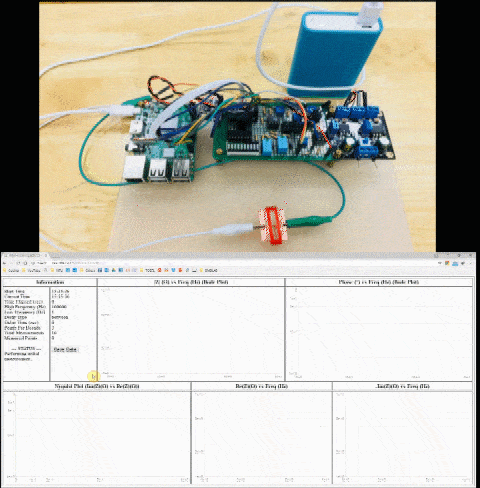## Real-time Impedance Detection System β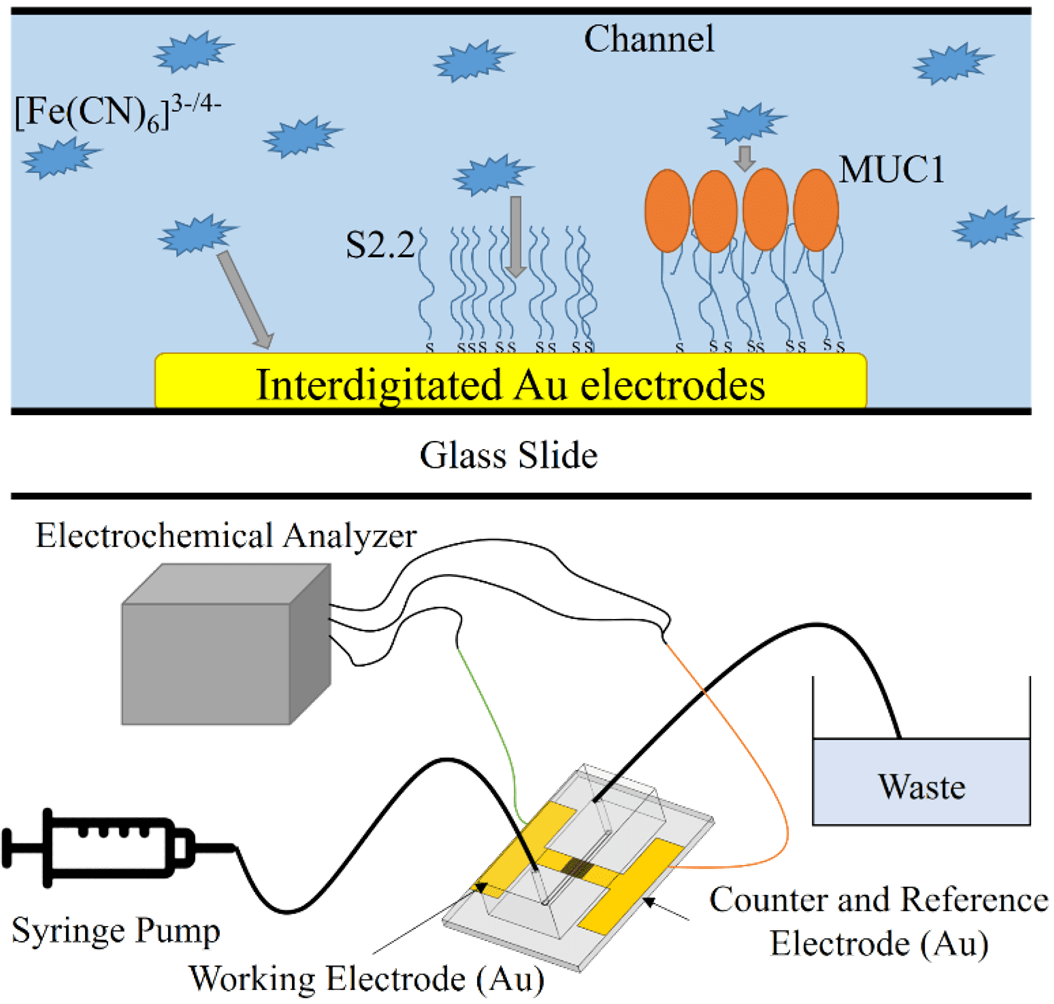## Real-time Microfluidic Impedimetric Aptasensing System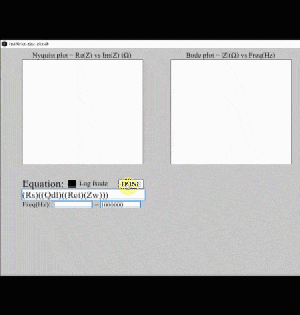## Electrochemical Impedance Modeling Programs

I started to use a technique called electrochemical impedance spectroscopy (EIS) for biosensing since my undergraduate research. For analyzing EIS data, an equivalent circuit must be constructed for modeling the reaction mechanism. Physical parameters can be extracted by fitting the data using the model. However, for most software, the circuit elements being provided and their corresponding combined circuit couldn’t necessarily meet my needs for finding the physical parameters in a symmetric electrode system. Therefore, I developed a circuit fitting program for customized analysis of impedance data. The fitting and impedance calculation program are used in a first-author journal paper of mine. In the following paragraphs, the development of the fitting program, another program that assists data visualization, and a program for calculating the diffusion impedance of interdigitated array (IDA) electrodes are detailed.

Figure 1. (a) The Nyquist plot for visualizing impedance values, and (b) an equivalent circuit with several circuit elements for modeling electrode surface reactions.

Electrochemical Impedance Circuit Fitting Program

The algorithm for finding all the element parameters in a given circuit is by implementing the Levenberg-Marquardt non-linear fitting method. This is a general and popular method for solving the minimum value of function E(x), where

$$E(x)={1 \over 2} \sum_{i=1}^m [f_i(x)]^2$$

For impedance data fitting, a complex non-linear least square process (CNLS) is implemented, and the above equation can be specialized as

$$S=\sum_{i=1}^{N_f} [w_{i,\mathrm{Re}}( \mathrm{Re}(Z_{i,cal}) – \mathrm{Re}(Z_{i,exp}) )^2+w_{i,\mathrm{Im}}( \mathrm{Im}(Z_{i,cal}) – \mathrm{Im}(Z_{i,exp}) )^2]$$

where S is the weighted sum of squares of error, Nf is the number of frequencies within an experiment, Zi is the impedance of the i–th frequency, and wi,Re and wi,Im are the statistical weights for the real and imaginary parts of the impedance of the i–th frequency. The subscript exp indicates experimental value and the subscript cal indicates the calculated value while fitting.

For any kind of circuit, Zi,cal can be calculated by the set of element parameters and the frequency (e.g. R for a resistor, C and f for a capacitor), then S can be calculated using its defined equation. By minimizing S, the fitted element parameters can be modified so that the result impedance (Zi,cal) can be as close as possible to the experimental value (Zi,exp). Fig. 2 shows an example for a non-linear curve fitting process.

Figure 2. A non-linear curve fitting process (source: https://en.wikipedia.org/wiki/Gauss%E2%80%93Newton_algorithm).

A program written in C language is designed using the open-source numerical analysis library ALGLIB® to implement numerical integrations and calculations. ALGLIB is also used for non-linear least squares fitting of EIS data using Levenberg-Marquardt method. Several circuit elements for EIS data fitting are available in this program, which are shown in Table 1. Detailed methods for calculating the IDA diffusion element is shown in my first-author paper.

Table 1. Available circuit elements in the fitting program.

A circuit description code is defined to express equivalent circuits. Elements, including whole blocks inside parentheses, are either in series or parallel with each other. Those inside an odd number of pair of parentheses are in parallel with each other, and those inside an even number of pair of parentheses are in series. Fig. 3 shows a circuit equivalent to the description code of “R(RC)(R(RC))”.

Figure 3. Circuit description code and its corresponding circuit. Equivalent parts are marked by identical colored blocks.

Figure 4. Snapshot of the equivalent circuit fitting program.

Real-time Impedance Plotting Program

It is reasonable that an element in a circuit contributes to the impedance change to a certain degree. For instance, a resistor has an influence on the real part of impedance, and a capacitor affects its imaginary part. However, when the circuit consists of elements with serial or parallel combinations, it can be quite difficult to imagine how the shape of impedance data would change according to each element.

Therefore, I wrote a program for plotting impedance data in real-time using Processing language. After entering the circuit description code into the program, a Nyquist plot, total impedance Bode plot, and phase angle bode plot is generated. The user can use horizontal bars to control the value of a specific element. By changing its value, the impedance would be calculated immediately, and the plot would be drawn out in real-time.

Figure 5. User interface for the real-time impedance plotting program.

Here’s a clip for demonstrating the real-time impedance plotting program.

IDA Diffusion Impedance Calculation Program

The IDA diffusion element is a novel element for parameterizing the diffusion impedance of an IDA electrode using its shape factor (we/w), magnitude of the admittance (Y0), and the dimensionless frequency (w2ω/D). However, complex functions are required for calculating its value, such as the Bessel function, the complete elliptic integral of the second kind, and definite integrals.

Due to calculation difficulties, a program is written for calculating the IDA diffusion impedance. The program consists of two files “settings.txt” and “IDA_diff_Z.exe”. “settings.txt” is of the format below:

These contain all the 12 parameters for the usage of this program. A detailed description is listed in order below:

1. If [output to txt file] is 0, then calculated impedances will be shown directly on screen. If it is 1, then the values will be saved to the file “Z_diffusion_IDA.txt”.
2. The maximum frequency for impedance calculation can be set after [max freq (Hz)].
3. The minimum frequency for impedance calculation can be set after [min freq (Hz)].
4. The number of frequency points within a decade can be set (e.g. If [points per decade] is set to 5, then frequencies calculated between 101 and 100Hz will be 101, 100.8, 100.6, 100.4, 100.2 and 100Hz.)
5. we can be set after [electrode bandwidth we (um)].
6. wg can be set after [gap width wg (um)].
7. l can be set after [electrode length l (mm)].
8. N can be set after [number of bands N].
9. D can be set after [diffusion coefficient D (m^2/s)].
10. C* can be set after [bulk concentration C* (mM)].
11. n can be set after [number of electrons n].
12. T can be set after [temperature T (K)].

After setting the 12 parameters, put “settings.txt” and “IDA_diff_Z.exe” in the same directory and open “IDA_diff_Z.exe”. Impedances will be automatically calculated and printed in the defined format (Fig. 6). ※The program can only be run in a Windows 64-bit environment.

Figure 6. An example output of the IDA diffusion impedance calculation program.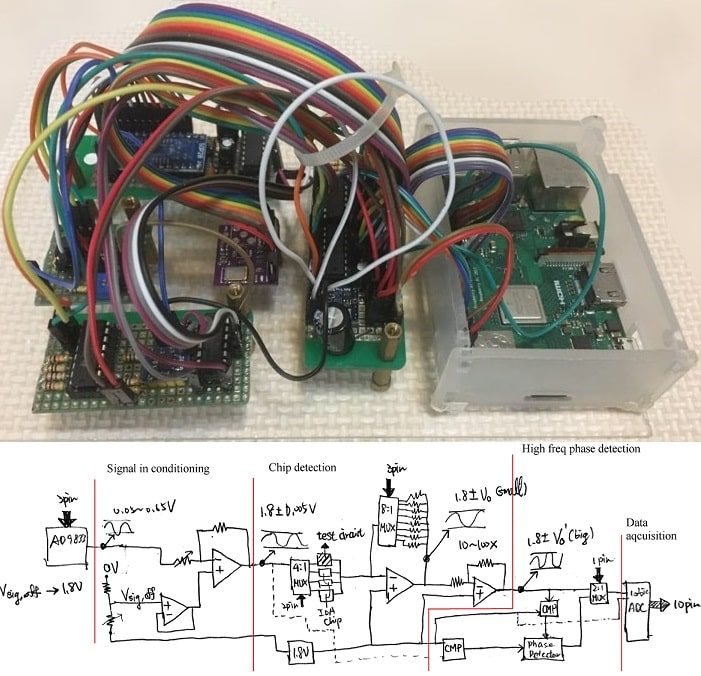## Real-time Impedance Detection System γ## Equivalent Circuit Impedance Plotting Program